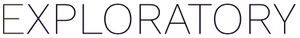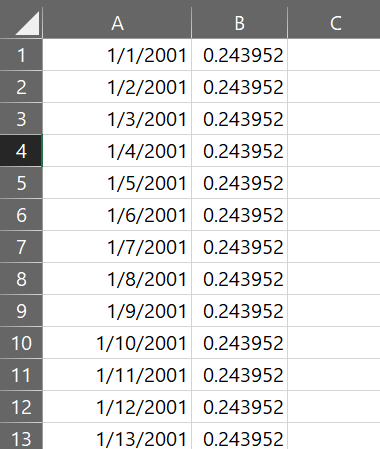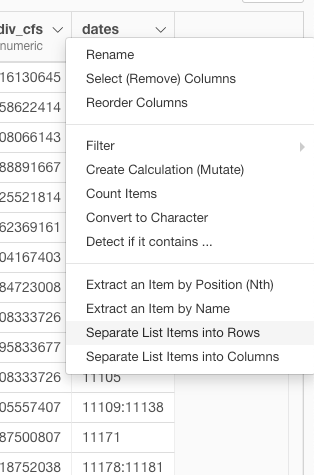# How to create a new data frame using seq()?

#1

Hello, we have a data frame that looks like this:

I would like to create a new data frame that has one row for each day, and the “water_use_per_day” column is repeated X times, where X= “NumDays”, as shown below:Thinking the `seq()` function might be helpful here, but my first attempts aren’t working out. Thanks for reading!

#2

Stephanie.

You are on the right track! Here is an example of how to use `seq` for generating sequences of dates in the R Script Data Frame.

``````data.frame(x= seq(as.Date("2020/1/1"), as.Date("2020/1/15"), by = "day"))
``````

1 Like
#3

Hi Kei!

Your suggestion and a bit more searching helped me to get this far:

But that is only for the first row. How can I make this work for the additional 232 rows?
Is there a way to do a custom command here?

#4

I think I misunderstood the question. Now I got the point. It is a bit complicated, but here is how.

You can create a new calculation with the following expression.

``````purrr::map2(date_time, num_days,
function(x, y){ seq(x, by="days", length.out = y)})
``````

It will create a list column like this. Each list contains a number of dates specified by “num_days” starting from “date_time”.

You can choose “Separate List Items into Rows” from the column header menu to expand those lists into rows.Then you will see the output like the following.